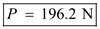# The coefficients of friction are µs = 0.40 and µk = 0.30 between all surfaces of contact. Determine the smallest force P required to start the 30-kg block moving if cable AB(a) is attached as shown, (b) is removed.

Question-AnswerCategory: Engineering MechanicsThe coefficients of friction are µs = 0.40 and µk = 0.30 between all surfaces of contact. Determine the smallest force P required to start the 30-kg block moving if cable AB(a) is attached as shown, (b) is removed.

The coefficients of friction are µs = 0.40 and µk = 0.30 between all surfaces of contact. Determine the smallest force P required to start the 30-kg block moving if cable AB(a) is attached as shown, (b) is removed.Step: 1

Given
Coefficient of static friction,Coefficient of kinetic friction,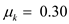Step: 2

Let us draw the free-body diagram of the system.Step: 3

a)
First apply the equilibrium condition,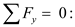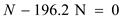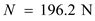Now,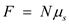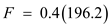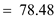Apply the equilibrium condition,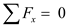Step: 4

Let us draw the free-body diagram of the second block.Step: 5

Apply the equilibrium condition,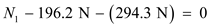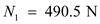Now,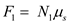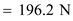Step: 6

Apply the equilibrium condition,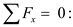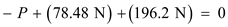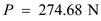Therefore, the force isStep: 7

b)
Without the cable, both blocks will stay together and motion will impend only at the floor.
Now, apply the equilibrium condition,Now,Step: 8

Apply the equilibrium condition,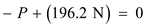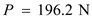Therefore, the force is